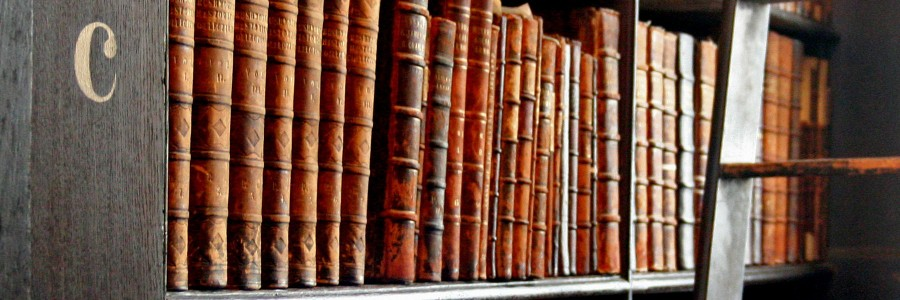# JFNK Preconditioning for coupled BWR calculations

C. Demazière, S. González-Pintor, A. Ålund.Proceedings of the PHYSOR 2018, Cancun, Mexico, Cancun, Mexico, 22-26 April 2018.

## Abstract

In this paper, a one-dimensional Boiling Water Reactor (BWR) model in steady-state conditions is developed. The model accounts for neutron transport, fluid dynamics, heat transfer and the interdependences between the various physics. The modelling assumptions were chosen so that the physics of BWRs could be properly accounted for with an as simple as possible model. The model is thereafter used to study the effect of preconditioning when a Jacobian-Free Newton Krylov (JFNK) method is used to solve the system of equations. Preconditioning is a key aspect in any JFNK method. By clustering the eigenvalues of the system of equations, a preconditioner is expected to drastically reduce the number of iterations. Nevertheless, the design of an efficient preconditioner is a far from trivial task. Using the developed model, different preconditioning alternatives are tested. It is demonstrated that an efficient preconditioner is found to have the following characteristics: separate preconditioning of the neutron transport problem, no preconditioning of the heat transfer problem, preconditioning of the fluid dynamics problem where the cross-dependencies between the void fraction and the phasic velocities are resolved and where the pressure field is treated independently. This paper thus provides guidelines about how to develop an efficient preconditioner for coupled BWR physics.